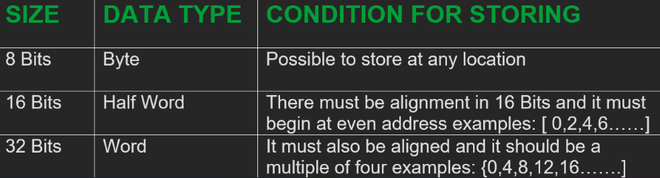Open in App
Not now

# Data Types in Advanced RISC Machine ( ARM7) Architecture

• Last Updated : 15 Sep, 2022

ARM is a processor architecture. It can be used for either microprocessor or microcontroller. The one in context here in our article is ARM7TDMI which is a microcontroller.

### Types of Data of Advanced RISC Machine(ARM 7)

ARM7 supports three data types. One is of 8 bits of data, the second is of 16 bits of data and the third is of 32 bits of data. ARM7 is 32 bits processor where 32 bits of data is referred to as a one-word size of data. So obviously with 16 bits, we can say that it is having a size of half-word 16 bits, and with 8 bits it is having a size of 1 byte. So that is how three data types are there in it.

There are a few essential conditions that must be considered while storing data into the memory, and while loading it from the memory inside the ARM processor.

### Storing Conditions

Word size with ARM7 is 32 bits and for half word, it has to be 16 bits. Here, while storing this data inside memory, a few essential conditions must be taken care of.  If we need to store data size with 8 bits, then it can be stored directly at any memory location with a byte but when it comes to the half-word size of data which is 16 bits at a time, it must be aligned and must be starting from even address, so we have one data of 16 bits, it be stored at starting memory location address [0,2,4,6,8….]. It should be stored in this way only as this is the correct way.

The reason for storing it at an even address is that when the ARM is reading this data or when the ARM is loading this data from the memory at that time if it is not aligned then it needs to have two machine cycles, but that is not going to happen ARM do not support data. It will take only one machine cycle to read that and for that, it should be stored with respect to even addresses for 16 bits. When it is having 32 bits of data size then for alignment, we will have to store this 4-byte data at a memory location which is a multiple of four. For example that address can be [0,4,8,12,16…].

If it is in multiple of 2, 4 then only ARM7 can read that data with a single machine cycle therefore these are the essential conditions.

When ARM7 is loading this stored data at that time the data will get stored inside the resistor. ARM 7 uses 32 bits of data resistor to store data. Now, if we have 8 bits of data then what we will be doing with 32 bits of the resistor? So for this, we need to extend those 8 bits to32 bits, and those extended bits can be stored inside the resistor. Similarly, for 16 bits of data, we need 16 bits for extension, and for 32 bits no bit is required for extension.  After loading, data from memory location data may have a size of 8 bits, 16 bits, or 32 bits but that data must be extended into 32 bits as it must be copied into a resistor, and the size of the resistor is 32 bits.### Extension of Data Types

Unsigned number: If there is an unsigned number then just insert 0’s ahead of the number and make that number 32 bits. For unsigned numbers, an extension can be done by just inserting 0s ahead of numbers.

Signed number: For a signed number we need to check whether the number is positive or negative.

• For a positive number, we will have to extend by inserting 0’s ahead of the number. For example, If Data = +7 = 0000 0111b then extension of this will be 0000 0000 0000 0000 0000 0000 0000 0111b.
• If the number is negative then insert one ahead of the number. For negative numbers, all computer systems is following 2’s complement to represent negative numbers. For example, If Data = -7 = 1111 1001b then extension of this will simply be 1111 1111 1111 1111 1111 1111 1111 1001b. For one’s complement just replace 0’s into 1’s and 1’s into 0’s; plus 1 that will make it to 2’s complement.

In binary for sign number, MSB shows the polarity of data. So, the number with MSB 1 for the signed number is negative and MSB with 0 is positive. Almost all of the computers follow negative number representation with two’s complement.

ARM supports load-store architecture therefore in single instruction we can load data from the memory into the resistor or we can store data of the resistor in memory.

My Personal Notes arrow_drop_up
Related Articles